# Scanner - Producer Equilibrium (2009-2018) Notes | Study Economics Class 11 - Commerce

## Commerce: Scanner - Producer Equilibrium (2009-2018) Notes | Study Economics Class 11 - Commerce

The document Scanner - Producer Equilibrium (2009-2018) Notes | Study Economics Class 11 - Commerce is a part of the Commerce Course Economics Class 11.
All you need of Commerce at this link: Commerce

N.C.E.R.T Questions

(Q1) Show that a perfectly competitive firm maximizes its profit only when Price = MC?

(Q2) What is meant by producer equilibrium ?

(Q3) What is condition of producer equilibrium or profit maximising for a competitive firm ?

(Q4) What is general condition of producer equilibrium or profit maximising of a firm ?

(Q5-6) Find out max profit by comparing MR and MC for (a) and (b) part. What is market price for
(c) part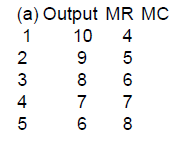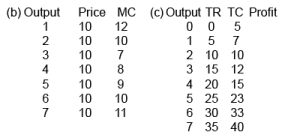(Q7) What condition must hold good if a profit - maximising firm produces positive output in a competitive market ?

(Q8) Can there be a positive level of output that a profit-maximising firm produces in a competitive market at which market price is not equal to marginal cost ? Explain

(Q9) Will a profit- maximising firm in a competitive market ever produce a positive level of output in the short run if the market price is less than minimum of AVC ? Give an explanation

(Q10) Will a profit- maximising firm in a competitive market ever produce a positive level of output in the range where the marginal cost is falling ? Give an explanation

(Q11) “A producer will increase his profit by reducing production when his MC is greater than his MR.” Explain.

(Q12) In a state of equilibrium . price greater than MC is ruled out for a perfectly competitive firm. Show diagrammatically

CBSE 2009

(Q1) The price of commodity X is Rs. 20 per unit and it remains constant. Given below is the cost schedule of one of its producers. Find out the level of output at which this producer is in equilibrium. Give reasons for your answer.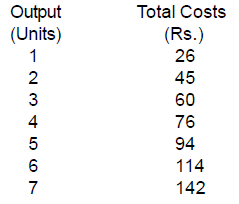(Q2) Find out the level of output at which this producer is in equilibrium. Give reasons for your answer.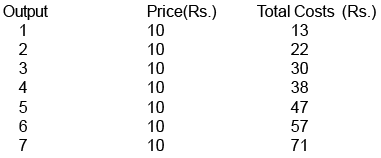CBSE 2010 + Sample Paper Questions

(Q1) From the following schedule find out the level of output at which the producer is in equilibrium,using marginal cost and marginal revenue qpproach. Give reasons for your answer.  (6M)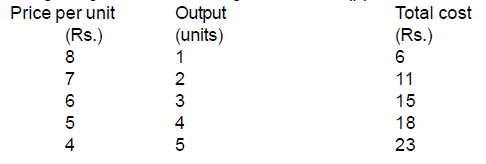(Q2) On the basis of the information given below, determine the level of output at which the producer will be in equilibrium. Use the marginal cost – marginal revenue approach. Give reasons for your answer.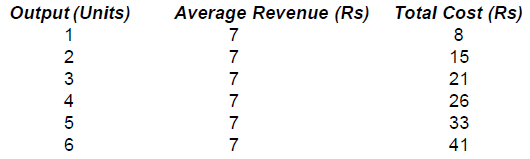CBSE 2011 & 2012

(Q1) What is producer’s equilibrium ? Explain the conditions of producer’s equilibrium through the ‘marginal cost and marginal revenue’ approach. Use diagram. (6 marks)

OR

What is producer’s equilibrium ? Explain the conditions of producer’s equilibrium through the ‘marginal cost and marginal revenue’ approach. Use a schedule. (6 marks)

C.B.S.E & Sample Paper 2013

(Q1) From the following table, find out the level of output at which the producer will be in equilibrium.Give reasons for your answer.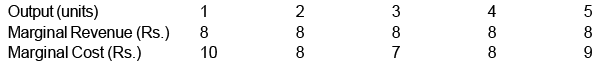(Q2) Explain the conditions of equilibrium of a firm based on marginal revenue. Use diagram.(6 M)

C.B.S.E Paper 2014

(Q1) From the following information about a firm, find the firm’s equilibrium output in terms of marginal cost and marginal revenue. Give reasons. Also find profit at this output.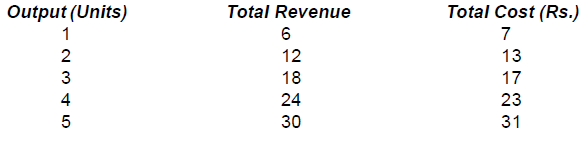(Q2) Using MC-MR method in the following table, find out the level of output at which the producer is in equilibrium. Give reasons for your answer. (4 marks)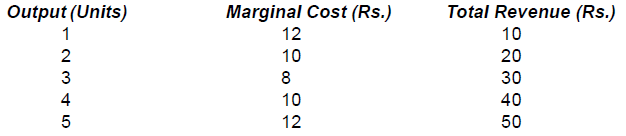Sample Paper 2015

(Q1) Explain the condition of equilibrium of a firm based on marginal cost and marginal revenue.

(6 M)

Extra Numerical

Using MC-MR method in the following tables, find out the level of output at which the producer is in

(Q1)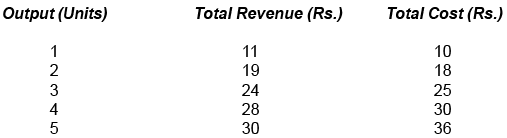(Q2)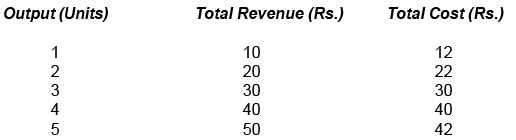(Q3) Determine producer’s equilibrium from the following data through MC-MR approach . Give reasons for your answer.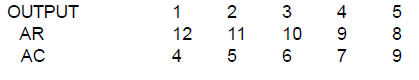C.B.S.E Paper 2015

(Q1) Explain why will a producer not be in equi. if the conditions of equilibrium are not met.(6M)

(Q2) Elaborate the implication of the conditions of equilibrium of a firm.(4M)

(Q3) Give the meaning of producers equilibrium. A producer produces that quantity of his product at which marginal cost and marginal revenue are equal. Is he earning maximum profits ? Give reasons for your answers.

(Q4) Why is the equality between marginal cost and marginal revenue necessary for a firm to be in equilibrium? Is it sufficient to ensure equilibrium? Explain.

(Q5) Explain the rationale behind the conditions of equilibrium of a producer.

CBSE paper 2017

(Q1) Explain the conditions of producer’s equilibrium with the help of a numerical example. Use marginal cost and marginal revenue approach?

(Q2) From the following data find out the level of output that will give the producer maximum profit (use marginal cost and marginal revenue approach). Give reasons for your answer. (6M)

Output (units)             1         2          3          4       5

Total Cost (Rs.)           9        17        24        29     36

Total Revenue (Rs.)   11       20       27        32      35

Ans: 4th unit of output & Profit = 3

This conditions of producer’s equilibrium are :

(1) MC = MR

(2) Beyond the level of output at which MC = MR, MC must be greater than MR   (2M)

The producer is in equilibrium when he produces 4 units as at this level of output both the

conditions of producer’s equilibrium are satisfied.  (1M)

(Q3) From the following total cost and total revenue schedule of a firm, find out the level of output, using marginal cost and marginal revenue approach, at which the firm would be in equilibrium. Give reasons for your answer.                                (6M)

Output (units)              1        2       3      4            5

Total Revenue (Rs.)   16     30     42     52         60

Total Cost (Rs.)           14     27     39     49         61

Q4)  Given below is the cost schedule of a product produced by a firm. The market price per unit of the product at all levels of output is Rs. 12. Using marginal cost and marginal revenue approach, find out the level of equilibrium output. Give reasons for your answer :

Output (Units)                 1         2           3           4           5          6

Average Cost (Rs.)         12         11         10         10         10.4     11

(Q5) Using marginal cost and marginal revenue approach, find out the  level of output at which producer will be in equilibrium. Give reasons for your answer.

Output (Units)                1         2        3           4            5           6

Average Revenue (Rs.)  20      20     20         20         20         20

Total Cost (Rs.)              22      42      60         76         96         120

(Q6) Using marginal cost and marginal revenue approach, find out the  level of output at which producer will be in equilibrium. Give reasons for your answer.

Price                              6      6      6     6     6

Output (Units)             1       2     3     4      5

Total Cost (Rs.)           20    15     21   28   37

CBSE paper 2018

(Q1) Explain the conditions of producer’s equilibrium in terms of marginal revenue and marginal cost.

(Q2) Explain the conditions of producer’s equilibrium under perfect competition.    (4M)

The document Scanner - Producer Equilibrium (2009-2018) Notes | Study Economics Class 11 - Commerce is a part of the Commerce Course Economics Class 11.
All you need of Commerce at this link: CommerceUse Code STAYHOME200 and get INR 200 additional OFF

## Economics Class 11

201 videos|195 docs|64 tests

Track your progress, build streaks, highlight & save important lessons and more!

,

,

,

,

,

,

,

,

,

,

,

,

,

,

,

,

,

,

,

,

,

;